# Statistics

Scilab provides tools to perform data analysis and modeling.

## Descriptive statistics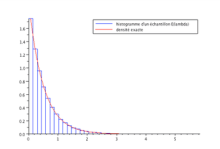median — median (row median, column median,…) of vector/matrix/array entries
stdev — standard deviation (row or column-wise) of vector/matrix entries
variance — variance (and mean) of a vector or matrix (or hypermatrix) of real or complex numbers

## Probability distributions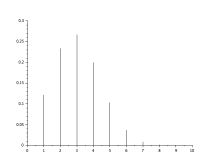Example with the binomial distribution probabilities: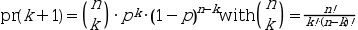```n=10;p=0.3;
plot2d3(0:n,binomial(p,n));```

More on wikipedia

## Linear and nonlinear modeling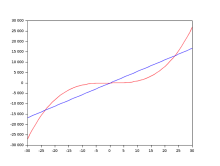The simplest example is a Linear regression:

```x = -30:30; y = x.^3;
[a, b] = reglin(x, y);
plot(x, y, "red")
plot(x, a*x+b)```

## Machine learning

Scilab provides tools to perform predictive analytics and machine learning for both:

• supervised learning
• unsupervised learning

Supervised learning is a most common kind of machine learning approach. It basically works with a set of “right answers” that is being used for the learning phase.

Unsupervised learning on the opposite does not require to have a training set a labeled data. It automatically identifies the hidden structure behind the dataset, and group them in a certain number of classes defined by the user.

## RegressionA regression problem deals with the prediction of a continuous function.

This example coming from the Scilab demos is a polynomial regression:

```0001  function y=FF(x)
0002      // parametric function model
0003      y = a*(x-b)+c*x.*x;
0004  endfunction

--> exec("SCI/modules/optimization/demos/datafit/datafit.dem.sce");
```

## Classification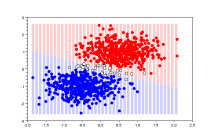A classification problem predicts discrete-valued outputs of a function.

This kind of problems can be addressed with support vector machines, implemented in the Scilab toolbox LibSVM.

Find out more with this tutorial in english and in french